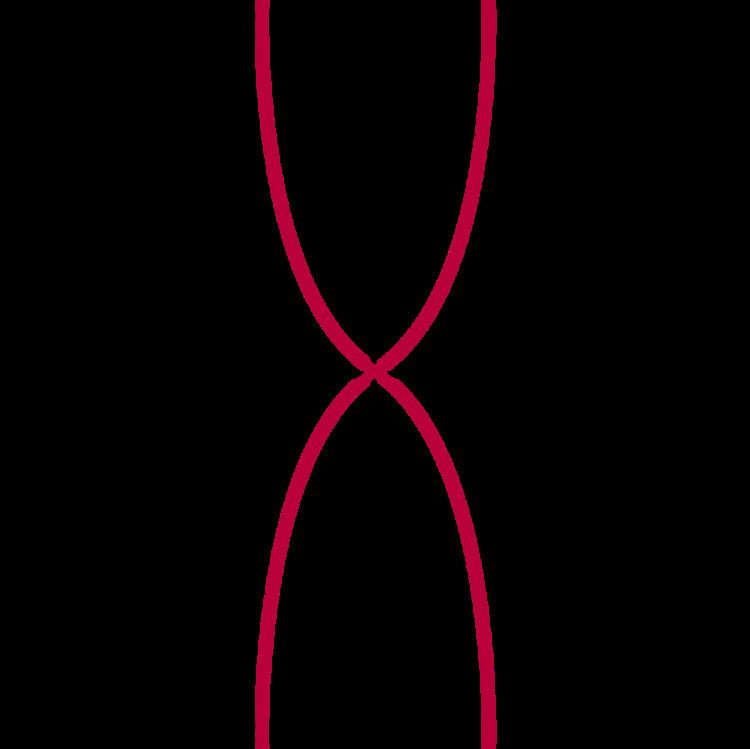# Bullet nose curve

Updated on
Covid-19In mathematics, a bullet-nose curve is a unicursal quartic curve with three inflection points, given by the equation

a 2 y 2 b 2 x 2 = x 2 y 2

The bullet curve has three double points in the real projective plane, at x=0 and y=0, x=0 and z=0, and y=0 and z=0, and is therefore a unicursal (rational) curve of genus zero.

If

f ( z ) = n = 0 ( 2 n n ) z 2 n + 1 = z + 2 z 3 + 6 z 5 + 20 z 7 +

then

y = f ( x 2 a ) ± 2 b

are the two branches of the bullet curve at the origin.

## References

Similar Topics
Mike Bassett: England Manager
Michael Aird